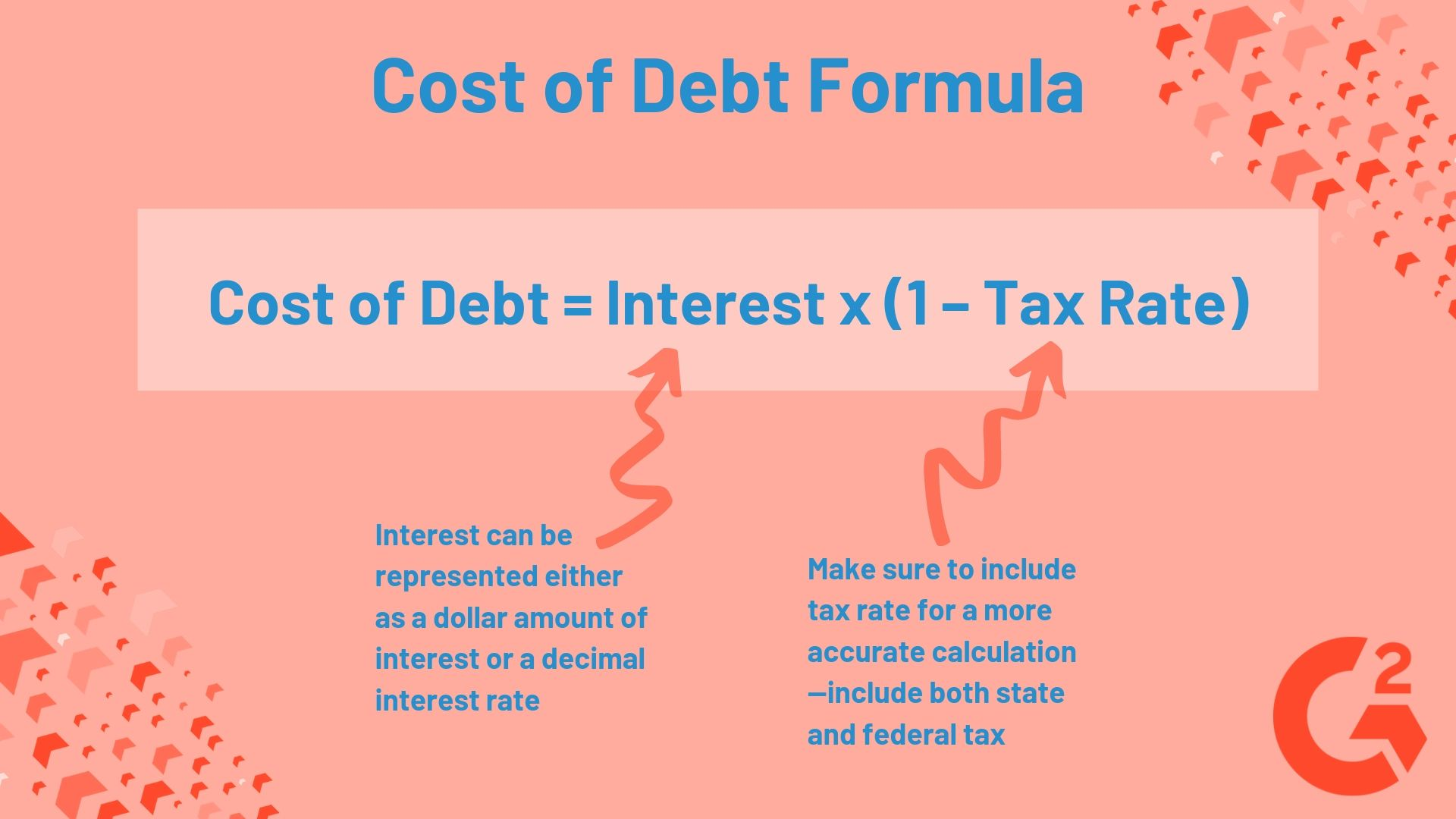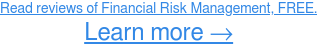Spontaneity makes life fun and interesting, but when it comes to finance, whims aren’t so great.

While a spur-of-the-moment trip to Italy or a random skydiving excursion might sound like a thrill, taking out a spontaneous loan is not a good idea.

Debt financing is an essential cog in the system of corporate capital structure, but business owners would be ill-advised to use it without determining what it’s going to cost them in the long run. This is when cost of debt comes into play. After interest and tax, how much does issuing bonds or taking out a term loan truly cost a company? Learn more about cost of debt and how to calculate it in this article.

# What is cost of debt?

Cost of debt is a representation of the debt a company owes its lenders. It is most commonly shown as a decimal expressing the rate of interest the company owes, but can also be expressed as an amount of money it owes in interest.

Finding cost of debt is also an element of determining a company’s weighted average cost of capital, or WACC, which not only accounts for the cost of debt, but the cost of equity as well, and is used to analyze the stability of a company’s capital structure.

You can think of cost of debt as the amount of interest paid on debt over a period of time, or the true cost of debt capital. Find your cost of debt using this formula:

As you can see, the cost of debt for a company not only includes interest, but also the company’s income tax rate. Typically, you’ll want to include the tax rate when calculating cost of debt because it will give you more accurate results.

 Tip: If you expect the tax rate on interest payments to change, you can calculate cost of debt without accounting for tax rate, or by substituting a projected tax rate in the formula.

Sometimes interest payments for companies in the U.S. are tax-deductible, so by factoring in the tax rate for those payments, your results will show a lower cost of debt.

## Why calculate cost of debt?

Both companies and investors use cost of debt to make financial decisions. Company officials look at cost of debt when they are deciding whether or not to take on more debt. If they know how much the debt will cost them, they can determine an ideal interest rate and if they can afford to take out a loan in the first place.

Investors use cost of debt to determine the default risk of investing in a company. When a company has a higher cost of debt, they are at higher risk of defaulting, or not being able to pay off the debt. If investors determine a high lending risk, they will offer higher interest rates to the company.Related: When it comes to corporate finance, debt isn't always a bad thing if it's managed appropriately. Discover how companies use a sinking fund to plan ahead and pay off their debts.

## Cost of debt examples

We can calculate cost of debt as either a decimal rate using a company’s interest rate, or a dollar amount of debt using a company’s total interest payments.

Use the decimal method when analyzing a company’s WACC, and dollar amount when you want to figure out how many dollars a company is spending on debt, either in all of its finances or for a single loan.

### Dollar amount

A small company takes out a term loan with a principal amount of \$50,000. The interest rate on the loan is 6% and the tax rate is 25%.

Before we can plug these numbers into the cost of debt formula, we have to find the total interest to be paid on the loan by multiplying the interest rate by the principal amount.

\$50,000 x 0.06 = \$3,000

Now that we know the total amount of interest to be paid on the loan is \$3,000, we can factor in the tax rate as a decimal:

\$3,000 x (1 – 0.25) = \$2,250

The total cost of debt for the loan is \$2,250 after taxes.

### Decimal

The same formula is used to determine the decimal cost of debt—simply use interest rate rather than a dollar amount of interest.

Let’s say a large corporation pays \$150,000,000 in annual interest on its \$4,000,000,000 of total debt, and is taxed at 35%. Before we can use this data to calculate the company’s cost of debt, we must find the interest rate it pays. To do this, divide the annual interest by the total debt, then multiply the product by 100.

(\$150,000,000/\$4,000,000,000) x 100 = 0.375

We have determined the company’s interest rate; now let’s plug it into the cost of debt formula, along with the tax rate (again, represented as a decimal):

0.375 x (1 – 0.35) = 0.24

The corporation’s cost of debt is 0.24, or 24%.